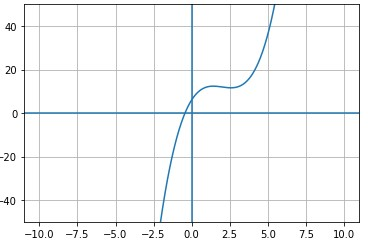# Determine the roots of: f(x)=x^{3}-6x^{2}+11x+6

## Question:

Determine the roots of: {eq}f(x)=x^{3}-6x^{2}+11x+6 {/eq}

## Finding roots of a function:

The roots of a function are those values which when substituted in the variables, satisfy the equation. Basically they are also the zeros of the polynomial.We can clearly see that the equation has only one real root as it crosses the {eq}x-axis{/eq} only once and rest it has two complex conjugate roots.

{eq}x^{3}-6x^{2}+11x+6=0{/eq}

Since the function is unsolvable by hit and trial methods,

We find it by a complicated formula or can find it from the graph and finally roots are

{eq}-0.434 , 3.217 + i* 1.856 , 3.217 - i* 1.856{/eq}